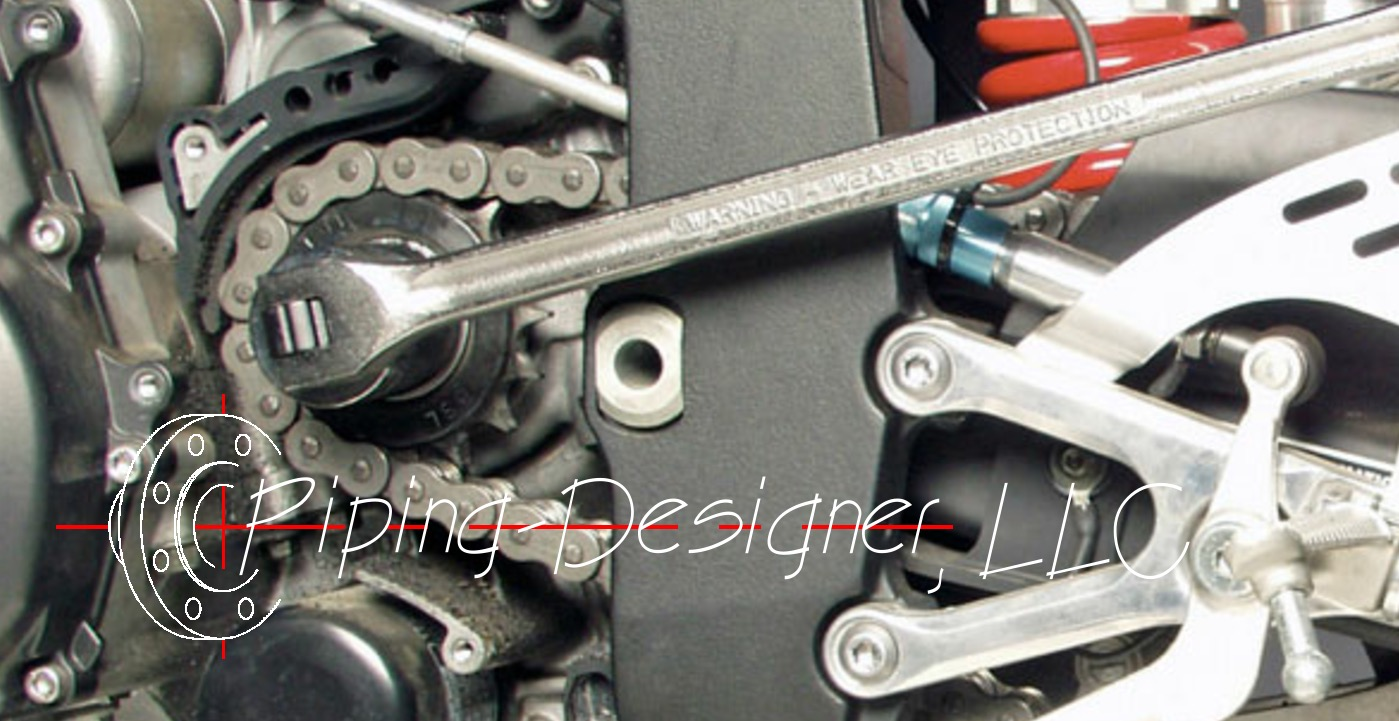# Torque

Written by Jerry Ratzlaff on . Posted in Classical MechanicsTorque, abbreviated as $$\tau$$ (Greek symbol tau) or T, also called moment of force, is a rotational moment.  it is a measure of how much twisting is applied to a fastener. The units used to measure torque are in the form of force times length. Usually measured in newton-metres (Nm) if metric units are used or pounds feet (lb-ft) when imperial units are used.

### Torque formula

(Eq. 1)  $$\large{ \tau = l \; F }$$

(Eq. 2)  $$\large{ \tau = d \; F }$$

(Eq. 3)  $$\large{ \tau = I \; \alpha }$$

Where:

$$\large{ \tau }$$  (Greek symbol tau) = torque

$$\large{ \alpha }$$  (Greek symbol alpha) = angular acceleration

$$\large{ d }$$ = distance

$$\large{ F }$$ = force

$$\large{ l }$$ = length, moment arm

$$\large{ I }$$ = moment of inertia

Solve for:

$$\large{ d = \frac {\tau}{F} }$$

(Eq. 1)  $$\large{ F = \frac {\tau}{l} }$$

(Eq. 2)  $$\large{ F = \frac {\tau}{d} }$$

$$\large{ l = \frac {\tau}{F} }$$

## Breakaway Torque

The torque necessary to put into reverse rotation a bolt that has not been tightened.

## Breakloose Torque

The torque required to effect reverse rotation when a pre-stressed threaded assembly is loosened.